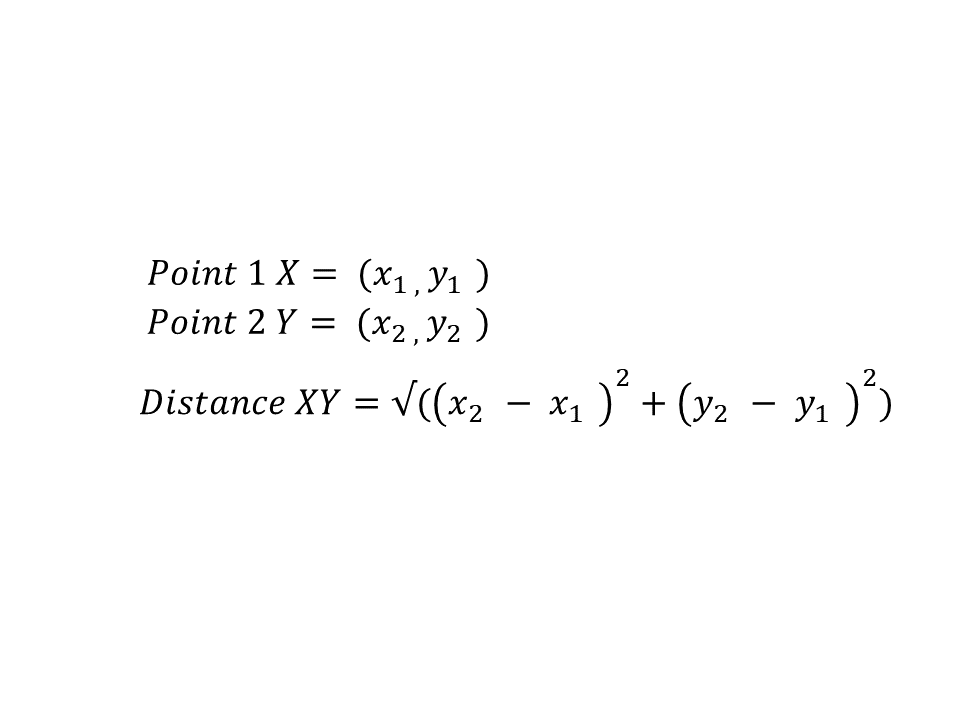# Calculate Distance Between Two Points in Java

Sheeraz Gul May 27, 2022

Using the Pythagorean theorem, we can find the distance between two points in Java. This tutorial demonstrates how to calculate the distance between two points in Java.

## Calculate Distance Between Two Points in Java

For example, the two points `X` and `Y` have the coordinates `(x1, y1)` and `(x2, y2)`, the distance between these two points can be denoted as `XY`, and the Pythagorean theorem can be implemented in Java to calculate the distance.

The equation in the picture below represents the Pythagorean theorem for these two points.Let’s try to implement the Pythagorean theorem in Java.

``````package delftstack;

import java.util.Scanner;
public class Distance_Two_Points {
public static void main(String[] args) {
Scanner Temp = new Scanner(System.in);

// declare the variables
int x1;
int x2;
int y1;
int y2;
int x;
int y;
double Distance_Result;

// get the input coordinates
System.out.print("Enter the values of first point coordinates : ");
x1 = Temp.nextInt();
y1 = Temp.nextInt();
System.out.print("Enter the values of second point coordinates : ");
x2 = Temp.nextInt();
y2 = Temp.nextInt();

// Implement pythagorean theorem
x = x2-x1;
y = y2-y1;
Distance_Result = Math.sqrt(x*x + y*y);

System.out.println("Distance between the two points is : " + Distance_Result);
}
}
``````

The code above will take the coordinates of two points and then calculate the distance by the Pythagorean theorem. See output:

``````Enter the values of first point coordinates : 12
21
Enter the values of second point coordinates : 13
34
Distance between the two points is : 13.038404810405298
``````
Author: Sheeraz Gul

Sheeraz is a Doctorate fellow in Computer Science at Northwestern Polytechnical University, Xian, China. He has 7 years of Software Development experience in AI, Web, Database, and Desktop technologies. He writes tutorials in Java, PHP, Python, GoLang, R, etc., to help beginners learn the field of Computer Science.